Get instant live expert help with Excel or Google Sheets“My Excelchat expert helped me in less than 20 minutes, saving me what would have been 5 hours of work!”

#### Post your problem and you’ll get expert help in seconds.

Your message must be at least 40 characters
Our professional experts are available now. Your privacy is guaranteed.

# Learn How to Use Fuzzy Lookup in Excel

We can use Excel Fuzzy Lookup Add-In to match similar, but not exactly matching data. FUZZY LOOKUP returns a table of matched similar data in the chosen column. FUZZY LOOKUP is useful for comparing two same data sets where one of them comes from an external source and can be misspelled or typed incorrectly. This article will guide all levels of excel users on how to use FUZZY LOOKUP.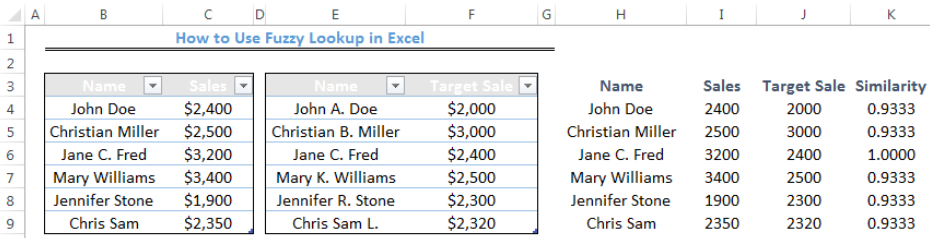Figure 1- How to Use Fuzzy Lookup in Excel

## Installation of Fuzzy Lookup in Excel

You won’t find FUZZY LOOKUP in the standard excel tabs and buttons. Download it by clicking HERE and follow the installation instructions.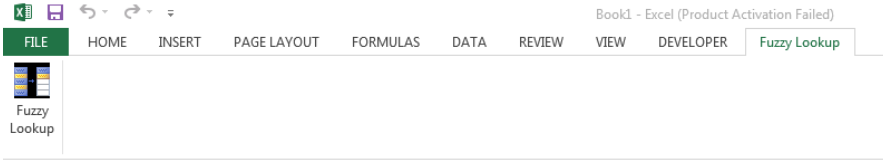Figure 2- Fuzzy Lookup Installed In Excel

## Creation of Fuzzy Lookup Table with the Data

We must format our data into tables to use Fuzzy Lookup.

• To do this, we will select the cell range for each table
• We will click on Insert tab and choose TableFigure 3- Creation of Fuzzy Lookup Table with the Data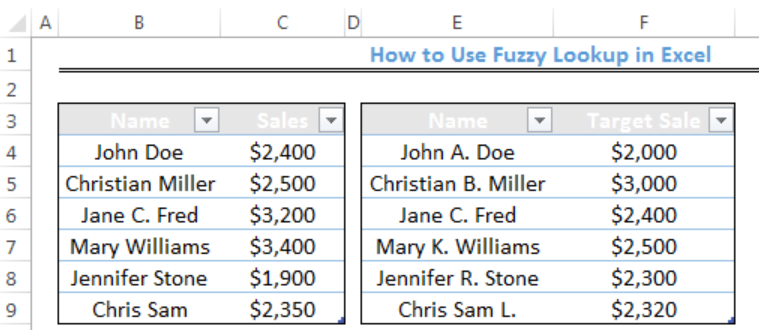Figure 4- Creation of Fuzzy Lookup Table with the Data

After we have created the two tables, we need to name them to enable their use by Fuzzy Lookup function. We do this by selecting the whole table and enter a name into the Name Box. The name box is where we have H5 in figure 5.

We will enter Sales as the name of the first table and Target Sales for the second table.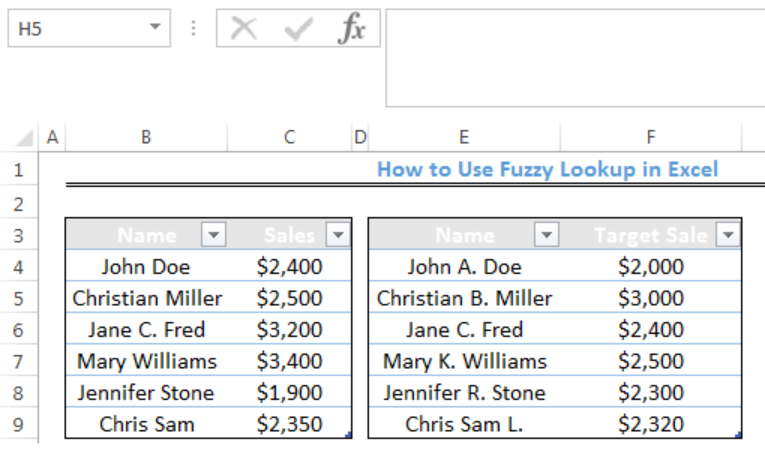Figure 5- Naming the tables for Fuzzy Lookup Use

## Using the FUZZY LOOKUP Function

Our aim is to use the fuzzy lookup function to match Name in the first table with Sales in the first table including target sales in the second table based on their similarity. We are assuming that the names in the second table aren’t spelled correctly.

• First, we will click on Cell H3 where our new table will begin
• We will click on Fuzzy lookup and click on Fuzzy Lookup below file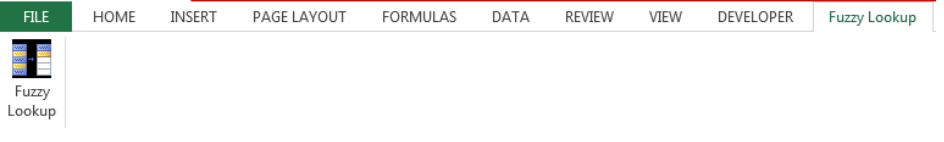Figure 6- Using the FUZZY LOOKUP Function

• For the output column, we will check the following boxes: Table1.Name, Table1.Sales, Table2.Target Sale, and FuzzyLookup.Similarity
• We will set the Similarity Threshold as 0.9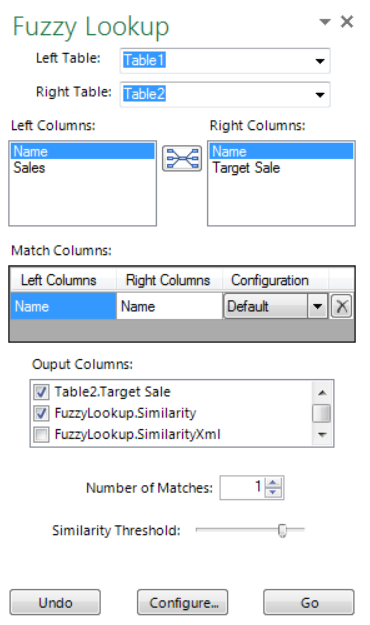Figure 7- Using the FUZZY LOOKUP Function

• We will click Go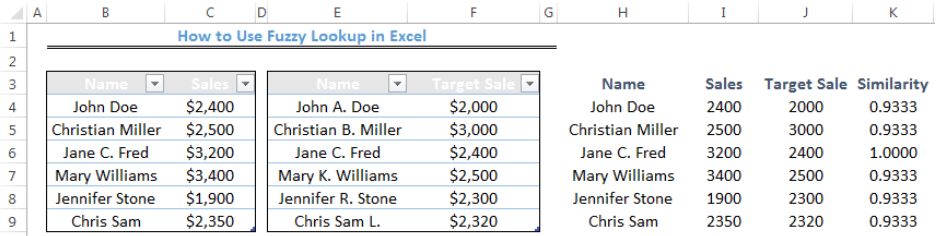Figure 8- Result of the Fuzzy Lookup Function

## Instant Connection to an Expert through our Excelchat Service

Most of the time, the problem you will need to solve will be more complex than a simple application of a formula or function. If you want to save hours of research and frustration, try our live Excelchat service! Our Excel Experts are available 24/7 to answer any Excel question you may have. We guarantee a connection within 30 seconds and a customized solution within 20 minutes.

Solution examplesI'm trying to figure out how to have a value found in column c placed into column d if the value in column a equals that found in column b. Does that make sense?
Solved by I. H. in 40 minsWhen i do a VLOOKUP, I cannot autofill the formula for the rest of the table. It copies the initial cell that the VLOOKUP was done.
Solved by G. A. in 20 minsI need a formula (I think VLOOKUP) that will enable me to: Use data from one column, compare with another and tell me the difference. Im a teacher and want to work out the amount of marks needed to the next grade boundary. I've created the rest, but this has me stumped!
Solved by F. A. in 28 minshow do I do the same formula for different cells, but i want a different cells value in each of the formulas. For example, if i was trying to find the percentage grade of each student in a class of 300.
Solved by I. W. in 14 minsI'm not sure as to why my formula isn't working. I will send two files... Workbook 1.xlsx.xls Workbook 2.xlsm The problem is in Column H of Workbook 1.
Solved by E. H. in 42 mins